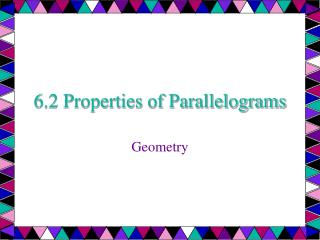DownloadDownload Presentation6.2 Properties of Parallelograms

# 6.2 Properties of Parallelograms

Download Presentation## 6.2 Properties of Parallelograms

- - - - - - - - - - - - - - - - - - - - - - - - - - - E N D - - - - - - - - - - - - - - - - - - - - - - - - - - -
##### Presentation Transcript

1. 6.2 Properties of Parallelograms Geometry

2. Objectives: • Use some properties of parallelograms. • Use properties of parallelograms in real-lie situations such as the drafting table shown in example 6.

3. Assignment: • Springboard Page 181 • Check your understanding e • Exercises: 1,4.5,6,7 and 8. • Use additional paper and • Show all your work. • Keep it in your book for • Further check it out.

4. In this lesson . . . And the rest of the chapter, you will study special quadrilaterals. A parallelogram is a quadrilateral with both pairs of opposite sides parallel. When you mark diagrams of quadrilaterals, use matching arrowheads to indicate which sides are parallel. For example, in the diagram to the right, PQ║RS and QR║SP. The symbol PQRS is read “parallelogram PQRS.”

5. 6.2—If a quadrilateral is a parallelogram, then its opposite sides are congruent. ►PQ≅RS and SP≅QR Theorems about parallelograms Q R P S

6. 6.3—If a quadrilateral is a parallelogram, then its opposite angles are congruent. P ≅ R and Q ≅ S Theorems about parallelograms Q R P S

7. 6.4—If a quadrilateral is a parallelogram, then its consecutive angles are supplementary (add up to 180°). mP +mQ = 180°, mQ +mR = 180°, mR + mS = 180°, mS + mP = 180° Theorems about parallelograms Q R P S

8. 6.5—If a quadrilateral is a parallelogram, then its diagonals bisect each other. QM ≅ SM and PM ≅ RM Theorems about parallelograms Q R P S

9. FGHJ is a parallelogram. Find the unknown length. Explain your reasoning. JH JK Ex. 1: Using properties of Parallelograms 5 G F 3 K H J b.

10. FGHJ is a parallelogram. Find the unknown length. Explain your reasoning. JH JK SOLUTION: a. JH = FG Opposite sides of a are ≅. JH = 5 Substitute 5 for FG. Ex. 1: Using properties of Parallelograms 5 G F 3 K H J b.

11. FGHJ is a parallelogram. Find the unknown length. Explain your reasoning. JH JK SOLUTION: a. JH = FG Opposite sides of a are ≅. JH = 5 Substitute 5 for FG. Ex. 1: Using properties of Parallelograms 5 G F 3 K H J b. • JK = GK Diagonals of a bisect each other. • JK = 3 Substitute 3 for GK

12. PQRS is a parallelogram. Find the angle measure. mR mQ Ex. 2: Using properties of parallelograms Q R 70° P S

13. PQRS is a parallelogram. Find the angle measure. mR mQ a. mR = mP Opposite angles of a are ≅. mR = 70° Substitute 70° for mP. Ex. 2: Using properties of parallelograms Q R 70° P S

14. PQRS is a parallelogram. Find the angle measure. mR mQ a. mR = mP Opposite angles of a are ≅. mR = 70° Substitute 70° for mP. mQ + mP = 180° Consecutive s of a are supplementary. mQ + 70° = 180° Substitute 70° for mP. mQ = 110° Subtract 70° from each side. Ex. 2: Using properties of parallelograms Q R 70° P S

15. PQRS is a parallelogram. Find the value of x. mS + mR = 180° 3x + 120 = 180 3x = 60 x = 20 Consecutive s of a □ are supplementary. Substitute 3x for mS and 120 for mR. Subtract 120 from each side. Divide each side by 3. Ex. 3: Using Algebra with Parallelograms P Q 3x° 120° S R

16. FURNITURE DESIGN. A drafting table is made so that the legs can be joined in different ways to change the slope of the drawing surface. In the arrangement below, the legs AC and BD do not bisect each other. Is ABCD a parallelogram? Ex. 6: Using parallelograms in real life

17. FURNITURE DESIGN. A drafting table is made so that the legs can be joined in different ways to change the slope of the drawing surface. In the arrangement below, the legs AC and BD do not bisect each other. Is ABCD a parallelogram? ANSWER: NO. If ABCD were a parallelogram, then by Theorem 6.5, AC would bisect BD and BD would bisect AC. They do not, so it cannot be a parallelogram. Ex. 6: Using parallelograms in real life